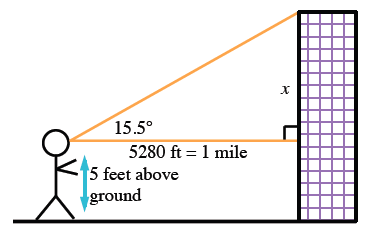### Home > INT2 > Chapter 5 > Lesson 5.2.5 > Problem5-116

5-116.

Kamillah decides to calculate the height of the Empire State Building. She walks $1$ mile ($5280$ feet) away from the tower and finds that she has to look up $15.5^\circ$ to see the top. Assuming Manhattan is flat, if Kamillah’s eyes are $5$ feet above the ground how tall is the Empire State Building?

Draw a diagram to help solve this problem.

$\text{tan}(15.5^\circ) = \frac{\textit{x}}{5280}$

Don't forget that $x$ is $5$ feet off the ground.